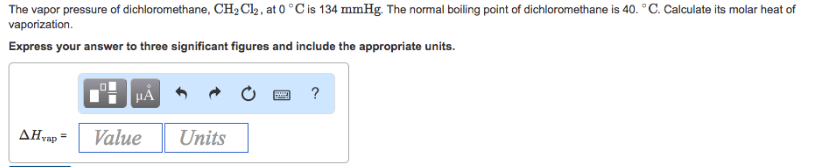# Problem: The vapor pressure of dichloromethane, CH 2C2, at 0°C is 134 g. The normal boiling point of dichloromethane is 40°C. Calculate its molar heat of vaporization. Express your answer to three significant figures and include the appropriate units.

###### FREE Expert Solution###### Problem Details

The vapor pressure of dichloromethane, CH 2C2, at 0°C is 134 g. The normal boiling point of dichloromethane is 40°C. Calculate its molar heat of vaporization.

Express your answer to three significant figures and include the appropriate units.